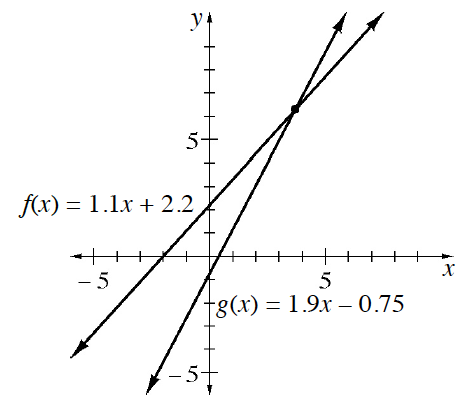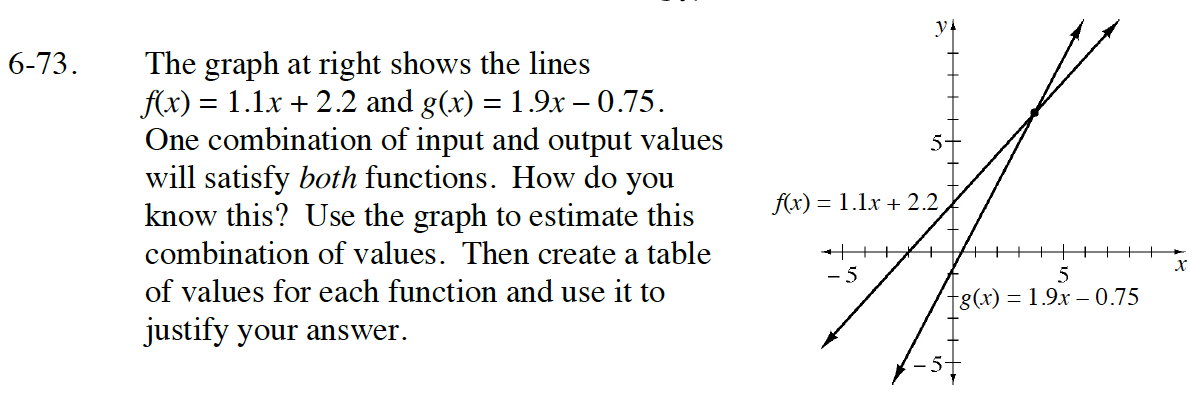Home > GB8I > Chapter cc46 > Lesson cc46.2.3 > Problem6-73

6-73.The graph at right shows the lines f(x) = 1.1x + 2.2 and g(x) = 1.9x – 0.75. One combination of input and output values will satisfy both functions. How do you know this? Use the graph to estimate this combination of values. Then create a table of values for each function and use it to justify your answer. Homework Help ✎What is the point at which the two lines intersect?

A reasonably close estimate is (3.5, 6) based solely on the provided graph.